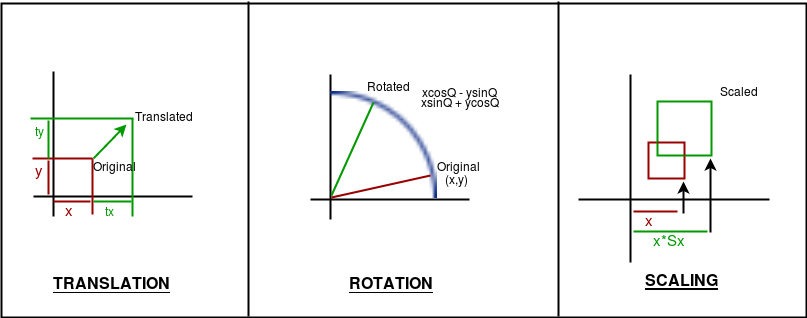GeeksforGeeks App
Open AppBrowser
Continue

# Basic Transformations in OPENGL

Transformations play a very important role in manipulating objects on the screen. It should be noted that here the algorithms will be implemented in code and the built-in functions will not be used to give a good understanding of how the algorithms work. Also, note that all transformations are implemented in 2D. There are three basic kinds of Transformations in Computer Graphics: 1. Translation 2. Rotation 3. ScalingAlgorithms:

1. Translation: Translation refers to moving an object to a different position on the screen.

```Formula:  X = x + tx
Y = y + ty
where tx and ty are translation coordinates

The OpenGL function is glTranslatef( tx, ty, tz );```

2. Rotation: Rotation refers to rotating a point.

```Formula:  X = xcosA - ysinA
Y = xsinA + ycosA,
A is the angle of rotation.
The above formula will rotate the point around the origin.
To rotate around a different point, the formula:
X = cx + (x-cx)*cosA - (y-cy)*sinA,
Y = cy + (x-cx)*sinA + (y-cy)*cosA,
cx, cy is centre coordinates,
A is the angle of rotation.

The OpenGL function is glRotatef (A, x, y, z). ```

3. Scaling: Scaling refers to zooming in and out an object on different scales across axes.

```Formula: X = x*sx
Y = y*sy,    sx, sy being scaling factors.

The OpenGL function is glScalef(float x, float y, float z)```

Note: If combined transformations are to be applied, follow the order: translate, rotate, scale

Implementation:

## C

 `// C code to implement basic``// transformations in OPENGL``#include ``#include ``#include ``#include `` ` `// Window size``#define maxWD 640``#define maxHT 480`` ` `// Rotation speed``#define thetaSpeed 0.05`` ` `// This creates delay between ``// two actions``void` `delay(unsigned ``int` `mseconds)``{``    ``clock_t` `goal = mseconds + ``clock``();``    ``while` `(goal > ``clock``());``}`` ` `// This is a basic init for the ``// glut window``void` `myInit(``void``)``{``    ``glClearColor(1.0, 1.0, ``                 ``1.0, 0.0);``    ``glMatrixMode(GL_PROJECTION);``    ``glLoadIdentity();``    ``gluOrtho2D(0.0, maxWD, ``               ``0.0, maxHT);``    ``glClear(GL_COLOR_BUFFER_BIT);``    ``glFlush();``}`` ` `// This function just draws ``// a point``void` `drawPoint(``int` `x, ``int` `y)``{``    ``glPointSize(7.0);``    ``glColor3f(0.0f, 0.0f, 1.0f);``    ``glBegin(GL_POINTS);``    ``glVertex2i(x, y);``    ``glEnd();``}`` ` `void` `rotateAroundPt(``int` `px, ``int` `py, ``                    ``int` `cx, ``int` `cy)``{``    ``float` `theta = 0.0;``    ``while` `(1) ``    ``{``        ``glClear(GL_COLOR_BUFFER_BIT);``        ``int` `xf, yf;`` ` `        ``// Update theta anticlockwise ``        ``// rotation``        ``theta = theta + thetaSpeed;`` ` `        ``// Check overflow``        ``if` `(theta>= (2.0 * 3.14159))``            ``theta = theta - (2.0 * 3.14159);`` ` `        ``// actual calculations..``        ``xf = (cx + (``int``)((``float``)(px - cx) * ``              ``cos``(theta)) - ((``float``)(py - cy) * ``              ``sin``(theta)));``        ``yf = (cy + (``int``)((``float``)(px - cx) * ``              ``sin``(theta)) + ((``float``)(py - cy) * ``              ``cos``(theta)));`` ` `        ``// Drawing the centre point``        ``drawPoint(cx, cy);`` ` `        ``// Drawing the rotating point``        ``drawPoint(xf, yf);``        ``glFlush();``       ` `        ``// Creating a delay``        ``// So that the point can be noticed``        ``delay(10);``    ``}``}`` ` `// This function will translate ``// the point``void` `translatePoint(``int` `px, ``int` `py, ``                    ``int` `tx, ``int` `ty)``{``    ``int` `fx = px, fy = py;``    ``while` `(1) ``    ``{``        ``glClear(GL_COLOR_BUFFER_BIT);`` ` `        ``// Update``        ``px = px + tx;``        ``py = py + ty;`` ` `        ``// Check overflow to keep ``        ``// point in screen``        ``if` `(px > maxWD || px < 0 || ``            ``py > maxHT || py < 0) ``        ``{``            ``px = fx;``            ``py = fy;``        ``}`` ` `        ``// Drawing the point``        ``drawPoint(px, py); `` ` `        ``glFlush();``       ` `        ``// Creating a delay``        ``// So that the point can be noticed``        ``delay(10);``    ``}``}`` ` `// This function draws``void` `scalePoint(``int` `px, ``int` `py, ``                ``int` `sx, ``int` `sy)``{``    ``int` `fx, fy;``    ``while` `(1) ``    ``{``        ``glClear(GL_COLOR_BUFFER_BIT);`` ` `        ``// Update``        ``fx = px * sx;``        ``fy = py * sy;`` ` `         ``// Drawing the point``        ``drawPoint(fx, fy); `` ` `        ``glFlush();``       ` `        ``// Creating a delay``        ``// So that the point can ``        ``// be noticed``        ``delay(500);`` ` `        ``glClear(GL_COLOR_BUFFER_BIT);`` ` `        ``// Update``        ``fx = px;``        ``fy = py;`` ` `        ``// Drawing the point``        ``drawPoint(fx, fy);``        ``glFlush();``       ` `        ``// Creating a delay``        ``// So that the point can be ``        ``// noticed``        ``delay(500);``    ``}``}`` ` `// Actual display function``void` `myDisplay(``void``)``{``    ``int` `opt;``    ``printf``(``"\nEnter\n\t<1> for translation"``           ``"\n\t<2> for rotation"``           ``"\n\t<3> for scaling\n\t:"``);``    ``scanf``(``"%d"``, &opt);``    ``printf``(``"\nGo to the window..."``);``    ``switch` `(opt){``    ``case` `1:``        ``translatePoint(100, 200, 1, 5);``        ``break``;``    ``case` `2:``        ``rotateAroundPt(200, 200, maxWD / 2, ``                       ``maxHT / 2);``         ` `        ``// Point will circle around``        ``// the centre of the window``        ``break``;``    ``case` `3:``        ``scalePoint(10, 20, 2, 3);``        ``break``;``    ``}``}`` ` `// Driver code``void` `main(``int` `argc, ``char``** argv)``{``    ``glutInit(&argc, argv);``    ``glutInitDisplayMode(GLUT_SINGLE | GLUT_RGB);``    ``glutInitWindowSize(maxWD, maxHT);``    ``glutInitWindowPosition(100, 150);``    ``glutCreateWindow(``"Transforming point"``);``    ``glutDisplayFunc(myDisplay);``    ``myInit();``    ``glutMainLoop();``}`